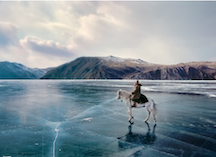# Will the ice crack?Ice has formed on a shallow pond and a steady state has been reached, with the air (above the ice) at $\displaystyle -5^o C$, and the bottom of the pond at $\displaystyle 4^o C$.

If the total depth of $\displaystyle \text{ice + water}$ is $\displaystyle 1.4m$, then find the thickness of the ice (in m) layer on the pond.

Details and Assumptions:
$\bullet$ $\displaystyle K_{ice} = 0.4 \text{cal}/m-^oC$
$\bullet$ $\displaystyle K_{water} = 0.125 \text{cal}/m-^oC$, where $\displaystyle K$ denotes the thermal conductivity.

Note: This problem appeared in our AITS (All India Test Series)

×

Problem Loading...

Note Loading...

Set Loading...